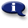# .

## Syllabus Information

Summer 2022
Dec 03,2023Use this page to maintain syllabus information, learning objectives, required materials, and technical requirements for the course.

Syllabus Information
MTH 085 - Applied Geometry for Technicians
Associated Term: Summer 2022
Learning Objectives: Upon successful completion of this course, the student will be able to:
1. Determine angle measurements in drawings involving triangles, parallel lines, and central angles
2. Use a protractor appropriately
3. Name basic shapes and describe their properties
4. Determine what folds up into a simple 3D shape
5. Apply properties of isosceles and equilateral triangles
6. Utilize the US Customary and metric system of units for length, area, volume, and weight
7. Use unit analysis to convert measurements including square and cubic units
8. Use conversion charts to convert measurements
9. Apply densities to determine weight or volume
10.Calculate perimeters, areas, volumes, and surface areas of geometric shapes
11.Evaluate formulas related to geometric measure
12. Solve applied ratio and proportion problems
13. Apply properties of similar triangles to find lengths
14. Apply properties of right triangles and use the Pythagorean Theorem
15. Apply trigonometric ratios to determine angles and lengths in right triangles
16. Apply the Law of Sines and the Law of Cosines (Optional)
17. Solve application problems involving geometry and measurement appropriate to technical fields
18. Simplify elementary algebraic expressions
19. Solve elementary algebraic equations and formulas
20. Communicate problem-solving steps clearly, particularly in graphics and writing
Required Materials:
Technical Requirements: